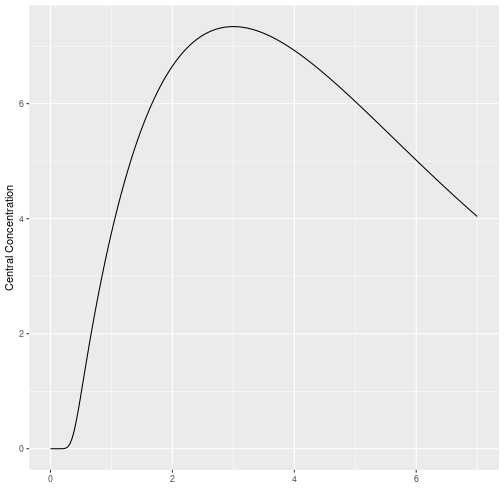<!–html_preserve–><!–/html_preserve–>

Savic 2008 first introduced the idea of transit compartments being a mechanistic explanation of a a lag-time type phenomena. RxODE has special handling of these models:

You can specify this in a similar manner as the original paper:

``````library(RxODE)
library(ggplot2)
mod <- RxODE({
## Table 3 from Savic 2007
cl = 17.2 # (L/hr)
vc = 45.1 # L
ka = 0.38 # 1/hr
mtt = 0.37 # hr
bio=1
n = 20.1
k = cl/vc
ktr = (n+1)/mtt
## note that lgammafn is the same as lgamma in R.
d/dt(depot) = exp(log(bio*podo)+log(ktr)+n*log(ktr*t)-ktr*t-lgammafn(n+1))-ka*depot
d/dt(cen) = ka*depot-k*cen
})

et <- eventTable();

transit <- rxSolve(mod, et, transit_abs=TRUE)

transit %>% ggplot(aes(time,cen))+geom_line()+ylab("Central Concentration")+
xlab("")
``````Another option is to specify the transit compartment function `transit` syntax. This specifies the parameters `transit(number of transit compartments, mean transit time, bioavailibility)`. The bioavailibity term is optional.

Using the `transit` code also automatically turns on the `transit_abs` option. Therefore, the same model can be specified by:

``````mod <- RxODE({
## Table 3 from Savic 2007
cl = 17.2 # (L/hr)
vc = 45.1 # L
ka = 0.38 # 1/hr
mtt = 0.37 # hr
bio=1
n = 20.1
k = cl/vc
ktr = (n+1)/mtt
d/dt(depot) = transit(n,mtt,bio)-ka*depot
d/dt(cen) = ka*depot-k*cen
})

et <- eventTable();
``````## Warning in rxSolve.default(mod, et): Assumed transit compartment model
``````transit %>% ggplot(aes(time,cen))+geom_line()+ylab("Central Concentration") +